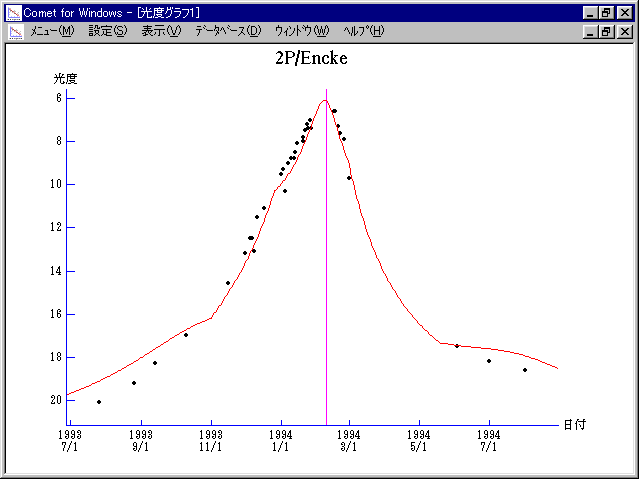# 2P/Encke (1994)###Magnitudes Graph

```        m1 = 15.0 + 5 log d +  5.0 log r  [    ,-100]  (             - 1993 Nov.  1)
m1 =  9.8 + 5 log d + 25   log r  [-100, -45]  (1993 Nov.  1 - 1993 Dec. 26)
m1 = 10.3 + 5 log d +  7.0 log r  [ -45,  20]  (1993 Dec. 26 - 1994 Mar.  1)
m1 = 12.3 + 5 log d + 15.7 log r  [  20, 100]  (1994 Mar.  1 - 1994 May  20)
m1 = 15.0 + 5 log d +  5.0 log r  [ 100,    ]  (1994 May  20 -             )
```##### The magnitudes graphs are made with Comet for Windows.